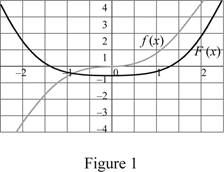# The value of the indefinite integral function ∫ x 3 x 2 + 1 d x .### Single Variable Calculus: Concepts...

4th Edition
James Stewart
Publisher: Cengage Learning
ISBN: 9781337687805### Single Variable Calculus: Concepts...

4th Edition
James Stewart
Publisher: Cengage Learning
ISBN: 9781337687805

#### Solutions

Chapter 5, Problem 36RE
To determine

## The value of the indefinite integral function ∫x3x2+1dx.

Expert Solution

The value of the indefinite integral is 13x2+1(x22)+C_

### Explanation of Solution

Given information:

The integral function is x3x2+1dx.

Consider (x2+1) as u.

u=x2+1 (1)

Differentiate both sides of the Equation (1).

du=2xdx (2)

Rearrange Equation (2) to find the value of x2 as shown below.

x2=u1 (3)

Substitute u for (x2+1), (u1) for x2, and 12du for xdx in the function as shown below.

x3x2+1dx=(u1)u(12du)=12(u11/21u)du=12(u1u)du (4)

The expression to find the indefinite integral value using Equation (4) as shown below.

x3x2+1dx=12(u1u)du=12(u12+1(12+1)u12+1(12+1))=12(u3/232u1/212)=u3/23u1/2 (5)

Substitute (x2+1) for u in Equation (5) as shown below.

x3x2+1dx=(x2+1)3/23(x2+1)1/2=(x2+1)1/2((x2+1)33)=13(x2+1)1/2(x22)=13x2+1(x22)+C

Therefore, the value of the indefinite integral is 13x2+1(x22)+C_.

Apply the Theorem:

The integral function f(x)dx is traditionally used for an antiderivative of f and is called an indefinite integral.

f(x)dx=F(x) (6)

Substitute x3x2+1dx for f(x) and 13x2+1(x22)+C for F(x) in Equation (6) as shown below.

x3x2+1dx=13x2+1(x22)+C (7)

Draw the graph for the function (f(x)) and its antiderivative (F(x)) using Equation (7) as shown below:Refer to Figure 1.

The function F(x) Increases with positive value of f(x) and F(x) decreases with negative value of f(x).

The minimum value of the function occurs at f(x)=0. Hence, it is reasonable from the graphical evidence.

Therefore, F(x) is an antiderivative of f(x).

### Have a homework question?

Subscribe to bartleby learn! Ask subject matter experts 30 homework questions each month. Plus, you’ll have access to millions of step-by-step textbook answers!